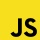Home
IT Knowledge
Inspiration
Languages
EN

# MS SQL Server - count if

0 points
Created by:Dirask JS Member
3610

In this article, we would like to show you how to count rows when the expression is true in MS SQL Server.

Quick solution:

``SELECT COUNT(CASE WHEN expresion THEN 1 END)``

Note:

Go to this article to read more about `CASE` statement.

## Practical example

To show you how `IF()` function works, we will use the following `users` table:

Note:

At the end of this article you can find database preparation SQL queries.

### Example

In this example, we will count the number of users whose salary is high, average or low.

Query

``````SELECT
COUNT(CASE WHEN [salary] = 6000 THEN 1 END) AS 'high_salary',
COUNT(CASE WHEN [salary] = 4000 THEN 1 END) AS 'average_salary',
COUNT(CASE WHEN [salary] = 2000 THEN 1 END) AS 'low_salary'
FROM [users];``````

Result:

## Database preparation

`create_tables.sql` file:

``````CREATE TABLE [users] (
[id] INT IDENTITY(1,1),
[name] VARCHAR(50) NOT NULL,
[surname] VARCHAR(50) NOT NULL,
[department_id] INT,
[salary] DECIMAL(15,2) NOT NULL,
PRIMARY KEY ([id])
);``````

`insert_data.sql` file:

``````INSERT INTO [users]
( [name], [surname], [department_id], [salary])
VALUES
('John', 'Stewart', 1, '6000'),
('Chris', 'Brown', 2, '6000'),
('Kate', 'Lewis', 3, '4000'),
('Ailisa', 'Gomez', NULL, '4000'),
('Gwendolyn', 'James', 2, '4000'),
('Simon', 'Collins', 4, '4000'),
('Taylor', 'Martin', 2, '2000'),
('Andrew', 'Thompson', NULL, '2000');``````